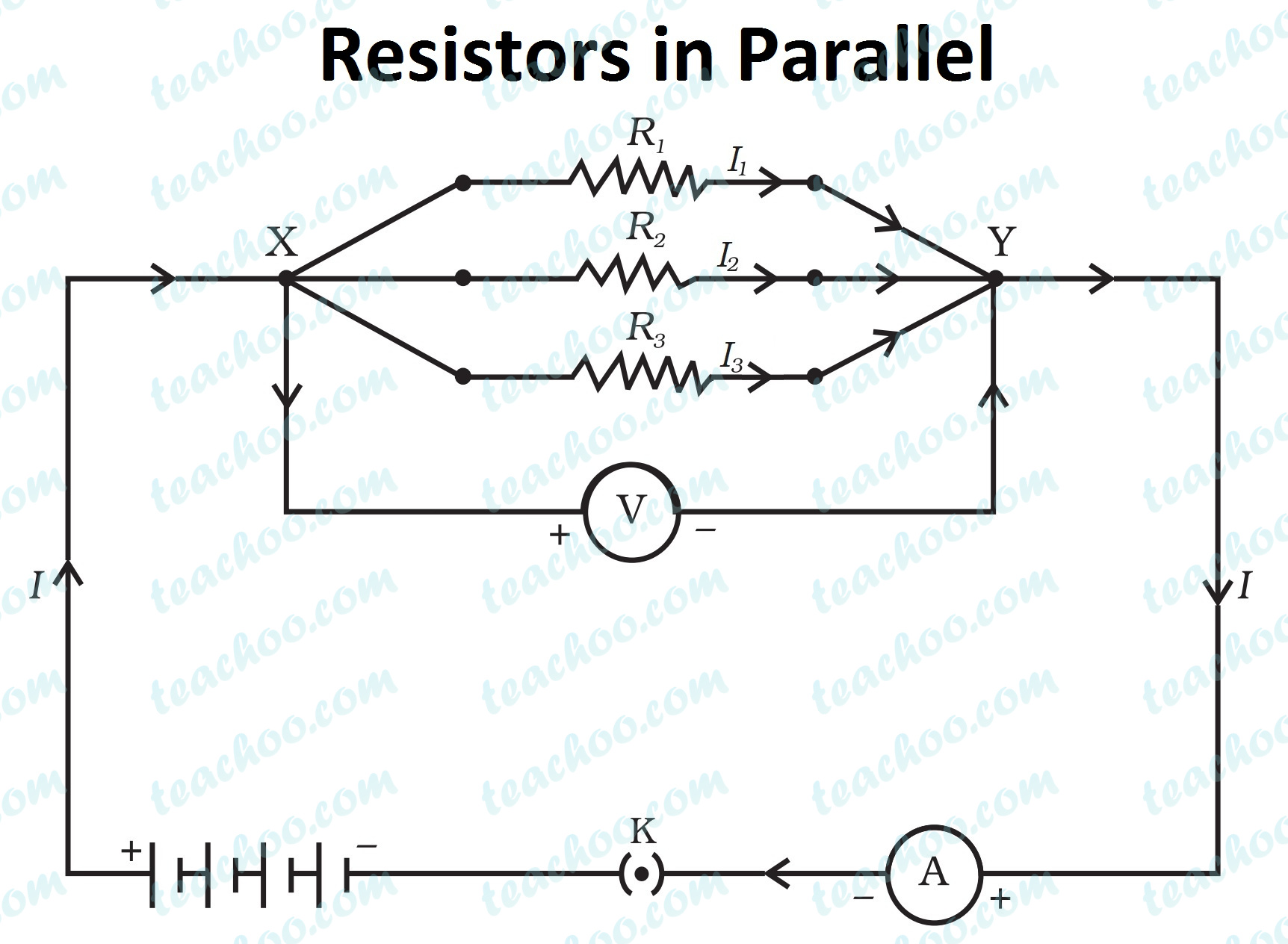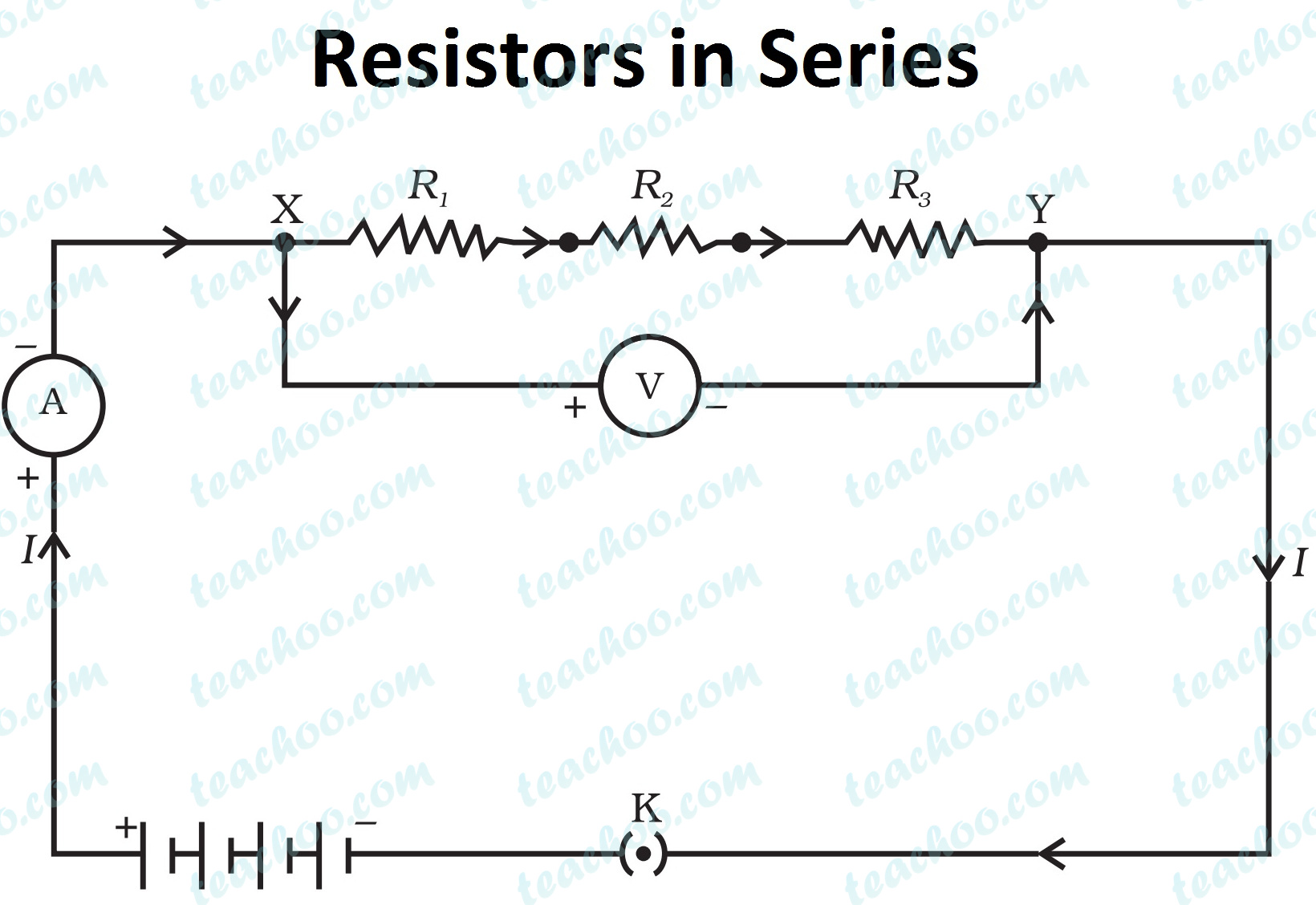Concepts

Class 10
Chapter 12 Class 10 - Electricity (Term 2)

## Electric Circuit in Series

• In this case, it is easier to switch on or off all the appliances connected together.
• Also it is safer as less current flows through circuit
• It is used when hundreds or thousands of bulbs are to be used together (So that same current passes through all, and they can switched on and off together.)
• Example-Diwali light decoration

• If one electrical appliance stops working due to some defect, then all other appliances would stop working.
• All electrical appliances have only one switch due to which they can not be turned on and off separately.
• All the appliances do not get the same voltage as the voltage gets divided in series combination.
• The equivalent resistance increases too much due to which the amount of current flowing becomes very small.

## Electric Circuit in Parallel

### Uses

It is normally used in homes for connecting bulbs and different household appliances

Each appliance has a separate switch which can be turned on or off as required

Even if one electrical appliance stops working due to some defect, all other appliances work fine.

Individual appliance can be turned off or on separately

Each electrical appliance gets the same voltage as the power supply line.

Since overall Resistance of Circuit decreases, devices can draw more current to operate

This method becomes cumbersome to use if thousands of bulbs/appliances are to be switched on or off

It is less safe as more current can pass through the circuit

## Differentiate between series and parallel combination of resistors.

 Series Parallel The current is same in all the resistors. The net current gets divided among individual resistors. The net voltage gets divided among individual resistors. The voltage is same across all resistors. Equivalent resistance is given by R = R 1 + R 2 + R 3 +... Equivalent resistance is given by 1/R = 1/R 1 + 1/R 2 + 1/R 3 +...## Questions

NCERT Question 18 (c) - Why is the series arrangement not used for domestic circuits?

Q3 Page 216 - What are the advantages of connecting electrical devices in parallel with the battery instead of connecting them in series?

641 students have Teachoo Black. What are you waiting for?